# Equations with one variable, the range of permissible values equation

Definition: an Equation with one variable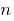is the equality with one variable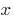, which in General form is written like this:Definition: a Root (or rozvyazka) equationis called the value of the variable that makes the equation true numerical equality.

Razvesti equation means finding all its roots (interchanges) or to show that they are not.

## The area of allowable values (odz) equation

Definition: the Area of allowable values (range of definition) of the equation — the overall scope for the functions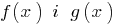standing in the left and right parts of the equation.

### Example

Set the equation: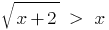DHS: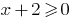i.e., as the domain of the function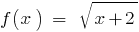is determined by the condition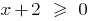, and the domain of the function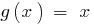is the set of all real numbers.

## Equations — investigation

If every root of first equation is the root of the second equation, the second equation is called the result of the first equation.

If the validity of the first equality implies the correctness of each of the following, then, adejumo equation is a consequence

Therefore, when using equations and effect verification of the roots by substitution in the original equation is a part of the solution.

### Example 1

Razvesti equation:Solution:

Let's build both parts of the equation in a square:;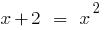;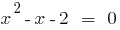;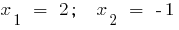.

Do a background check.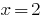— the root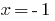is outside the root.

Answer: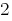.

## Equivalent equations

Definition: Equivalent (equivalent) equations — two equations, which for many DHS have the same outcome, that is, every solution of the first equation is rozvyazka the second, and Vice versa.

### Some theorems about equally dominant either equations

Theorem 1: If one part of the equation to move to another part of the terms with opposite sign, we get an equation equivalent to a given (on any set).

Theorem 2: If both parts of the equation be multiplied or divided by the same number not equal to zero (or to one and the same function that is defined and not equal to zero on the IDS of the given equation), we get equation equivalent to the given one.

Theorem 3: If both parts of the equationto take a rising (or descending) functionand not vdbase narrowing IDS of the given equationwill be equivalent to a given ( DHS).

## Corollaries of theorems about equally dominant either equations

Consequence: Because the function is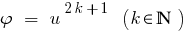monotonically increasing,then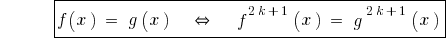.

In the presentation of both parts of the equation in odd natural degree of the resulting equation is equivalent to this.

Consequence: Because the function is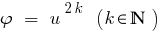monotonically increasing only if,in the case when both parts of the equation newmn, by lifting both parts even to the natural degree of the resulting equation is equivalent to this.

Tags:
Chapter:
Versions in other languages:
Share with friends: